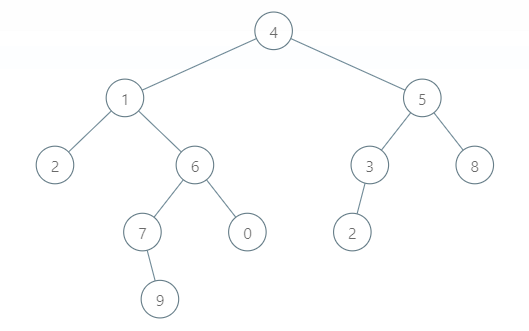# The Binary Lifting Technique

## What is the Binary Lifting Technique?

The Binary Lifting Technique is widely used to increase efficiency and subsequently reduce an algorithm’s time complexity. It is a faster algorithm and saves the computational power of the system.

There are many problems that we can solve with the Binary Lifting technique. Just to name a few:

• Finding the Lowest Common Ancestor in a Binary Tree in a logarithmic time.

• Finding minimum and maximum in a logarithmic time.

• Finding the sum of two nodes in a logarithmic time.

• Finding Kth ancestor of a node in a logarithmic time.

## The Lowest Common Ancestor Problem

The Lowest Common Ancestor of two nodes in a given binary tree is the lowest node that has both the given nodes as its descendants. Also, if one node is the descendant of the other node from the two nodes, then the parent node will be the Lowest Common Ancestor.For instance, in this given tree, the Lowest Common Ancestor of nodes 9 and 0 is 6 because 6 is the lowest ascendant of both 9 and 0.

The LCA of nodes 9 and 6 is 6 because 9 is the descendant of 6.

So, now that the problem is apparent, we’ll see the naive-brute force approach to solve this problem.

## Brute-Force Approach

The algorithm for a simple solution for this problem is:

• First, we’ll find the depth (distance from root node) of both nodes.

• Then we’ll bring the deeper node in the tree to the level of the other node. We’ll do this by making jumps of one (i.e., changing the node value to its parent value).

• When the two nodes are at the same level, if the values of both nodes are the same, we’ll return the value (this is the case when the other node itself is the LCA of both the nodes).

• We’ll keep jumping up both the nodes one by one till the value of both the nodes is not equal. (We can also compare the parent values of both nodes instead of their values)

• Finally, if the values of both nodes are the same, we’ll return the result.

This algorithm’s time complexity is O(n), and the space complexity of this brute-force approach is also O(n), where n is the number of nodes in the binary tree.

## Binary Lifting Approach

The problem with the above approach was that the time complexity was high O(n), So, let’s try to reduce the time complexity by using the Binary Lifting approach.

The idea of the Binary Lifting algorithm is that we reduce the number of upward jumps and hence reduce the number of comparisons.

In the brute force approach, we were making the jumps of one unit upwards and then comparing the values of both nodes, but now we’ll lift the nodes in the power of twos.

Here, Instead of making an upward jump of one, we’ll make a jump of the highest length j such that:

• j is a power of 2, and
• j is less than or equal to the difference between the depths of two nodes.

For instance, suppose the difference between the depths of two nodes is 14; according to the brute force solution, we’ll have to do a minimum of 13 comparisons.

But using Binary Lifting, we’ll bring down the number of comparisons down to 3.

When, d = 13 j = 8 (because 8 is the highest power of 2 less than d)

d = 5 j = 4

d = 1 j = 1

Hence, we can see that the time complexity is significantly reduced to O(logn) because we have reduced the number of comparisons from 13 to 3.

Input

Output

## Time Complexity

The time complexity of this code is O(logn), where n is the number of nodes in the Binary Tree.

## Space Complexity

The auxiliary space required by the algorithm is O(n), where n is the number of nodes in the Binary Tree or the size of the Binary Tree.

1). What is the Lowest Common Ancestor in a Binary Tree?

The Lowest Common Ancestor of two nodes in a given binary tree is the lowest node that has both the given nodes as its descendants.

2). What are the applications of finding LCA in a Binary Tree?

There are many use-cases of LCA in the programming; hence it becomes necessary to reduce the time complexity of this algorithm.

• In Computer Graphics, we use the LCA algorithm to find the smallest cube among two given cubes.
• In Object-oriented programming, we use LCA to find the most specialized superclass inherited by two different classes.
• In the Compiler design, we find the lowest common ancestor of two basic blocks. The basic computation can be inserted into the ancestor, and it is available for both blocks.

3). What is the time complexity of finding the LCA in a binary tree with Binary Lifting approach and What is the time required for preprocessing in Binary Lifting?

The worst-case, average-case and best-case time complexities of Binary Lifting approach for finding the LCA is O(logn), where n is the number of nodes in the Binary Tree.

The time complexity and the auxiliary space complexity of precomputing or preprocessing in binary lifting is O(n*logn).

## Key Takeaways

Today we learned what the Lowest Ancestor Problem is and how to devise an efficient solution using the Binary Lifting Technique.

If you are preparing for the upcoming Campus Placements, then don’t worry. Coding Ninjas has your back. Visit this link for a carefully crafted and designed course on-campus placements and interview preparation.